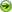Quiz MainSpace QuizMathematics QuizPhysics QuizBiology QuizChemistry QuizTechnology QuizAnimal Quiz

MATHEMATICS QUIZ

Do you feel mathematics is a subject which is to be feared hence avoided? Well try solving these mathematical sums given below and overcome your fear. Try solving it with concentration and surely you will succeed. And those who feel that solving mathematical problems is just an easy task well you should surely give it a try and enhance your merit to a higher extent. So what are you waiting for, go ahead and accept the challenge.
1. What is the next prime number after 7?

2.The perimeter of a circle is also known as what?

3. 65 – 43 = ?

4. True or false? A convex shape curves outwards.

5. What does the square root of 144 equal?

6. True or false? Pi can be correctly written as a fraction.

7. What comes after a million, billion and trillion?

8. 52 divided by 4 equals what?

9. What is the bigger number, a googol or a billion?

10. True or false? Opposite angles of a parallelogram are equal.

11. 87 + 56 = ?

12. How many sides does a nonagon have?

13. True or false? -2 is an integer.

14. What is the next number in the Fibonacci sequence: 0, 1, 1, 2, 3, 5, 8, 13, 21, 34?

15. 7 x 9 = ?

16. True or false? In an isosceles triangle all sides are unequal.

17. In statistics, the middle value of an ordered set of values is called what?

18. What does 3 squared equal?

19. True or false? -4 is a natural number.

20. 5 to the power of 0 equals what?

1. 11
2. The circumference
3. 22
4. True
5. 12
6. False
8. 13
9. A googol
10. True
11. 143
12. 9
13. True
14. 55
15. 63
16. False
(2 sides are equal)
17. The median
18. 9
19. False
20. 1

Back to Quiz Main